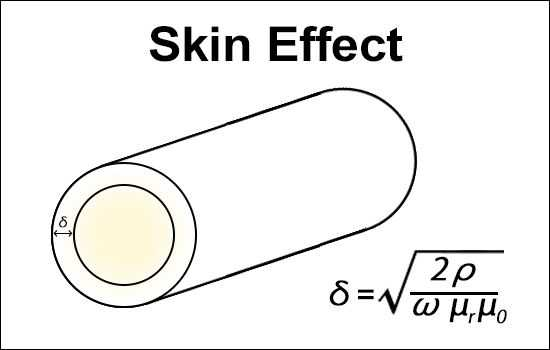# Skin Depth Calculator

This calculator calculates the skin depth resulting from the skin effect. The output is rounded to the fourth decimal place and is output in both "um" and "mils".### Input

f:

Resistivity of the conductor（Ω⋅m）:

×10-8

### Output

In the equation above, "ρ" is the resistivity of the conductor, "µr" is the magnetic permeability of the conductor, "µ0" is the permeability of free space(4π×10-7, and "ω" is the angular frequency.